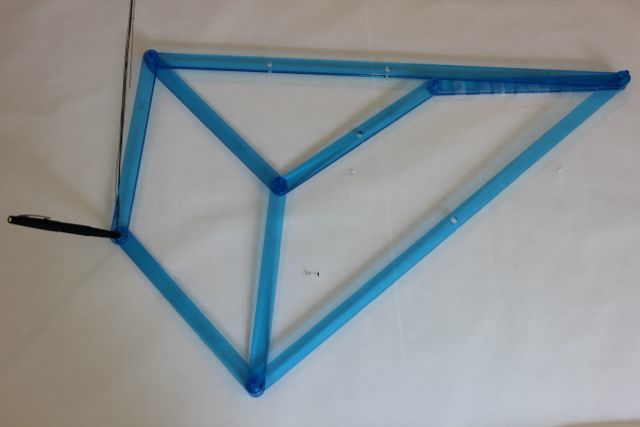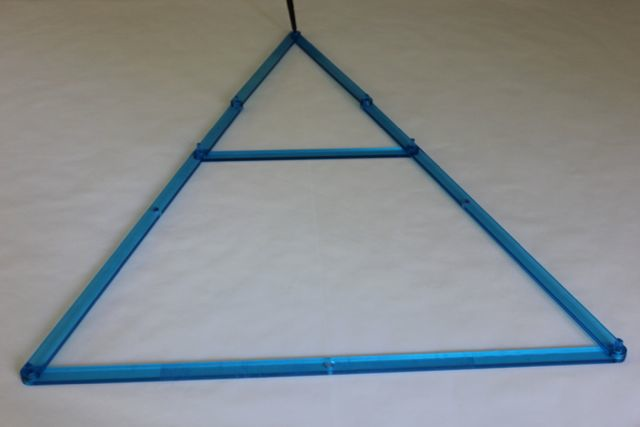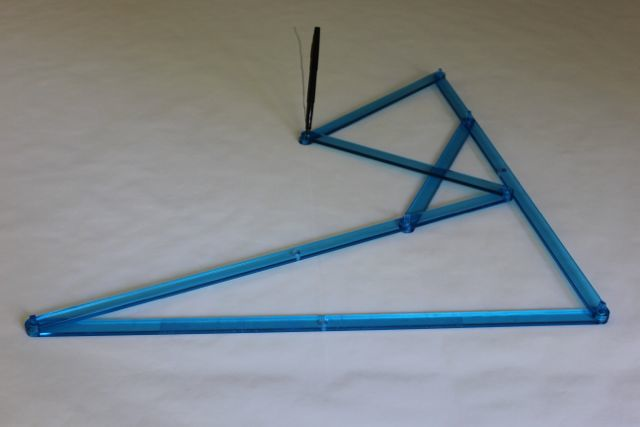Math Monday – Linkages: The Straight Dope

Math Monday: Linkages – The Straight Dope

by Glen Whitney

And here is yet another installment in our epic Math Mondays series on the intricate world of mechanical linkages. See the Linkages series introduction for the MoMath Linkage Kit, an introduction, and general instructions.

OK, the suspense surrounding the (non?)existence of a straight-line linkage has built long enough. Let’s cut to the chase:

Ingredients: Six 24-bars (A,B,C,D,F, and H), two 60-bars (B and D), and a pen.

Directions: Fix A horizontally. Link one end of A to B. Link the far end of B to both C and D. Link the far end of C to both E and F. Link the far end of D to G and H. Link the far ends of F and H with a pen. Link the far ends of E and G to the other end of A.

To use: Rotate B up and down as far as it will go, keeping the pen in the hole drawing on the paper.

And here are the pics:Is it… could it be… is that line the Peaucellier linkage is drawing an honest-to-goodness straight one? Indeed it is, and the reason pulls in some pretty cool mathematics: the theory of “inversion in a circle.” Inversion in a circle is a geometric operation which rearranges a plane in the following way: a point at a distance d from one special point (called the center of inversion) is moved to lie in the same direction from the center of inversion, but at a distance of 1/d. That’s all there is to it, but inversion in a circle has a number of interesting and useful properties: it preserves angles, and any curve which is either a circle or straight line is transformed into some other curve which is also either a circle or straight line. In particular, any circle which goes through the center of inversion is transformed into a straight line which does not go through the center of inversion. And that’s exactly the property Peaucellier needed: his linkage is essentially just a mechanism for performing inversion in a circle, connected to the very first, simplest linkage this series discussed: a compass, to draw the circle. In this case, the center of inversion is at the far end of A, and bar B describes a circle that goes through that center of inversion.

It’s also interesting to note that a student of Chebyshev’s, caught up in his advisor’s crusade to find a straight-line linkage, independently discovered the Peaucellier linkage about seven years later. It was an idea whose time had come, despite the fact that in practice with so many joints it is difficult to build a Peaucellier linkage that produces extremely accurate straight motion — there’s almost always a tiny bit of play in each joint, and it adds up.

Virtually any idea worth having can be refined, and this linkage is no exception. Here’s a linkage with significantly fewer joints and a very interesting motion. Note that as far as I know, there’s no connection between this and the prior author of Math Mondays.

Hart A-Frame
Ingredients: A 60-bar (A), two 60-bars with holes at 45 (B and C), three 30-bars (D, E, and F), and a pen.

Directions: Fix A horizontally. Link each end of A to a 60-bar with a hole at 45 (B and C). B to C0; link C30 to D and D back to the right end of A. Put a pen in C60. Link B45 to D and B60 to E. Link the other of end of D to C45. Link C60 to F, and link the free ends of E and F with a pen.

To use: Rotate B as far left and right as it will go, keeping the pen in the hole drawing on the paper.Can you work out why the Hart A-frame theoretically draws a perfectly straight line?

This article first appeared on Make: Online, December 17, 2012.